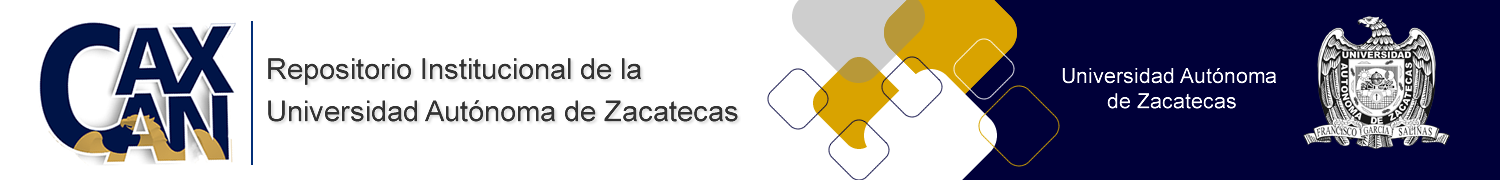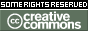Please use this identifier to cite or link to this item: http://ricaxcan.uaz.edu.mx/jspui/handle/20.500.11845/556
 Title: Is the free electromagnetic field a consequence of Maxwell´s equations or a postulate? Authors: 0000-0001-5324-1834 Issue Date: Dec-1998 Publisher: Springer Abstract: It is generally accepted that solutions of so called "free" Maxwell equations for Q = 0 (null charge density at every point of the whole space) describe a free electromagnetic field for which flux lines neither begin nor end in a charge). In order to avoid ambiguities and unacceptable approximation which have place in the conventional approach in respect to the free field concept, we explicitly consider three possible types of space regions: (i) uisolated charge-free" region, where a resultant electric field with the flux lines which either begin or end in a charge is zero in every point, for example, inside a hollow conductor of any shape or in a free-charge universe; (ii) "non-isolated charge-free" region, where this electric [see (i)] field is not zero in every point; and (Hi) "charge-neutral" region, where point charges exist but their algebraic sum is zero. According to these definitions a strict mathematical interpretation of Maxwell's equations gives following conclusions: (1) In "isolated charge-free" regions electric free field cannot be unconditionally understood neither as a direct consequence of Maxwell's equations nor as a valid approximation: it may be introduced only as a postulate; nevertheless, this case is compatible is the existence of a time-independent background magnetic field. (2) In both "charge-neutral" and "nonisolated charge-free" regions, where the condition Q = 6 function or g = 0 respectively holds, Maxwell's equation for the total electric field have non-zero solutions, as in the conventional approach.However, these solution cannot be strictly identified with the electric free field. This analysis gives rise to the reconsideration of the freeelectromagnetic field concept and leads to the simplest implications in respect to charge-neutral universe. URI: http://hdl.handle.net/20.500.11845/556 ISSN: 0894-9875 Other Identifiers: info:eu-repo/semantics/publishedVersion Appears in Collections: *Documentos Académicos*-- UA Física

This item is licensed under a Creative Commons License